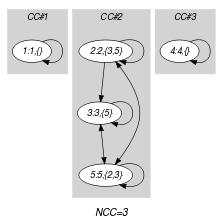## 5.205. k_cut

Origin

E. Althaus

Constraint

$𝚔_\mathrm{𝚌𝚞𝚝}\left(𝙺,\mathrm{𝙽𝙾𝙳𝙴𝚂}\right)$

Arguments
 $𝙺$ $\mathrm{𝚒𝚗𝚝}$ $\mathrm{𝙽𝙾𝙳𝙴𝚂}$ $\mathrm{𝚌𝚘𝚕𝚕𝚎𝚌𝚝𝚒𝚘𝚗}\left(\mathrm{𝚒𝚗𝚍𝚎𝚡}-\mathrm{𝚒𝚗𝚝},\mathrm{𝚜𝚞𝚌𝚌}-\mathrm{𝚜𝚟𝚊𝚛}\right)$
Restrictions
 $𝙺\ge 1$ $𝙺\le |\mathrm{𝙽𝙾𝙳𝙴𝚂}|$ $\mathrm{𝚛𝚎𝚚𝚞𝚒𝚛𝚎𝚍}$$\left(\mathrm{𝙽𝙾𝙳𝙴𝚂},\left[\mathrm{𝚒𝚗𝚍𝚎𝚡},\mathrm{𝚜𝚞𝚌𝚌}\right]\right)$ $\mathrm{𝙽𝙾𝙳𝙴𝚂}.\mathrm{𝚒𝚗𝚍𝚎𝚡}\ge 1$ $\mathrm{𝙽𝙾𝙳𝙴𝚂}.\mathrm{𝚒𝚗𝚍𝚎𝚡}\le |\mathrm{𝙽𝙾𝙳𝙴𝚂}|$ $\mathrm{𝚍𝚒𝚜𝚝𝚒𝚗𝚌𝚝}$$\left(\mathrm{𝙽𝙾𝙳𝙴𝚂},\mathrm{𝚒𝚗𝚍𝚎𝚡}\right)$ $\mathrm{𝙽𝙾𝙳𝙴𝚂}.\mathrm{𝚜𝚞𝚌𝚌}\ge 1$ $\mathrm{𝙽𝙾𝙳𝙴𝚂}.\mathrm{𝚜𝚞𝚌𝚌}\le |\mathrm{𝙽𝙾𝙳𝙴𝚂}|$
Purpose

Select some arcs of a digraph in order to have at least $𝙺$ connected components (an isolated vertex, i.e. a vertex without any ingoing or outgoing arc, is counted as one connected component).

Example
$\left(\begin{array}{c}3,〈\begin{array}{cc}\mathrm{𝚒𝚗𝚍𝚎𝚡}-1\hfill & \mathrm{𝚜𝚞𝚌𝚌}-\varnothing ,\hfill \\ \mathrm{𝚒𝚗𝚍𝚎𝚡}-2\hfill & \mathrm{𝚜𝚞𝚌𝚌}-\left\{3,5\right\},\hfill \\ \mathrm{𝚒𝚗𝚍𝚎𝚡}-3\hfill & \mathrm{𝚜𝚞𝚌𝚌}-\left\{5\right\},\hfill \\ \mathrm{𝚒𝚗𝚍𝚎𝚡}-4\hfill & \mathrm{𝚜𝚞𝚌𝚌}-\varnothing ,\hfill \\ \mathrm{𝚒𝚗𝚍𝚎𝚡}-5\hfill & \mathrm{𝚜𝚞𝚌𝚌}-\left\{2,3\right\}\hfill \end{array}〉\hfill \end{array}\right)$

The $𝚔_\mathrm{𝚌𝚞𝚝}$ constraint holds since the graph corresponding to the $\mathrm{𝙽𝙾𝙳𝙴𝚂}$ collection contains 3 connected components (i.e., two connected components respectively involving vertices 1 and 4 and a third connected component containing the remaining vertices 2, 3 and 5), and since the first argument $𝙺$ enforces to have at least 3 connected components.

Typical
$|\mathrm{𝙽𝙾𝙳𝙴𝚂}|>1$
Symmetries
• $𝙺$ can be decreased to any value $\ge 1$.

• Items of $\mathrm{𝙽𝙾𝙳𝙴𝚂}$ are permutable.

Keywords
Arc input(s)

$\mathrm{𝙽𝙾𝙳𝙴𝚂}$

Arc generator
$\mathrm{𝐶𝐿𝐼𝑄𝑈𝐸}$$↦\mathrm{𝚌𝚘𝚕𝚕𝚎𝚌𝚝𝚒𝚘𝚗}\left(\mathrm{𝚗𝚘𝚍𝚎𝚜}\mathtt{1},\mathrm{𝚗𝚘𝚍𝚎𝚜}\mathtt{2}\right)$

Arc arity
Arc constraint(s)
$\bigvee \left(\begin{array}{c}\mathrm{𝚗𝚘𝚍𝚎𝚜}\mathtt{1}.\mathrm{𝚒𝚗𝚍𝚎𝚡}=\mathrm{𝚗𝚘𝚍𝚎𝚜}\mathtt{2}.\mathrm{𝚒𝚗𝚍𝚎𝚡},\hfill \\ \mathrm{𝚒𝚗}_\mathrm{𝚜𝚎𝚝}\left(\mathrm{𝚗𝚘𝚍𝚎𝚜}\mathtt{2}.\mathrm{𝚒𝚗𝚍𝚎𝚡},\mathrm{𝚗𝚘𝚍𝚎𝚜}\mathtt{1}.\mathrm{𝚜𝚞𝚌𝚌}\right)\hfill \end{array}\right)$
Graph property(ies)
$\mathrm{𝐍𝐂𝐂}$$\ge 𝙺$

Graph model

$\mathrm{𝚗𝚘𝚍𝚎𝚜}\mathtt{1}.\mathrm{𝚒𝚗𝚍𝚎𝚡}=\mathrm{𝚗𝚘𝚍𝚎𝚜}\mathtt{2}.\mathrm{𝚒𝚗𝚍𝚎𝚡}$ holds if $\mathrm{𝚗𝚘𝚍𝚎𝚜}\mathtt{1}$ and $\mathrm{𝚗𝚘𝚍𝚎𝚜}\mathtt{2}$ correspond to the same vertex. It is used in order to enforce keeping all the vertices of the initial graph. This is because an isolated vertex counts always as one connected component. Within the context of the Example slot, part (A) of Figure 5.205.1 shows the initial graph from which we have chosen to start. It is derived from the set associated with each vertex. Each set describes the potential values of the $\mathrm{𝚜𝚞𝚌𝚌}$ attribute of a given vertex. Part (B) of Figure 5.205.1 gives the final graph associated with the example of the Example slot. The $𝚔_\mathrm{𝚌𝚞𝚝}$ constraint holds since we have at least $𝙺=3$ connected components in the final graph.

##### Figure 5.205.1. Initial and final graph of the $𝚔_\mathrm{𝚌𝚞𝚝}$ set constraint(a) (b)